# Binary Addition and Subtraction with Examples

In this tutorial, we will learn about the binary addition and subtraction with the help of examples. By Saurabh Gupta Last updated : May 10, 2023

Since binary numbers consist of only two digits 0 and 1, so their addition is different from decimal addition. Addition of binary numbers can be done following certain rules:

ABSumCarry
0000
0110
1010
1101

The above table contains two bits a and b, their sum and carry.

```    0 + 0 = 0,
0 + 1 = 1,
1 + 0 = 1,
1 + 1 = 10 (i.e., sum is 0 and carry is 1)
```

Let's do some exercise and solution some questions based on binary addition to get more of the topic.

### Example 1: Perform (10)2 + (11)2

#### Solution

Using the rules provided above, sum operation can be performed as: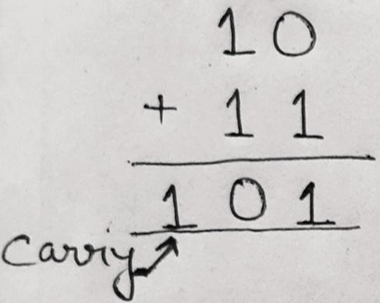Therefore, (10)2 + (11)2 = (101)2

Verification:

We can verify our result by converting the above binary numbers into decimal numbers and then verifying the sum.

Here, (10)2 = (2)10, (11)2 = (3)2 and (101)2 = (5)10, thus when we will add 2 and 3 we get sum as 5.

### Example 2: Perform (1)2 + (1)2 + (1)2 + (1)2

#### Solution

Using the rules provided above, sum operation can be performed as: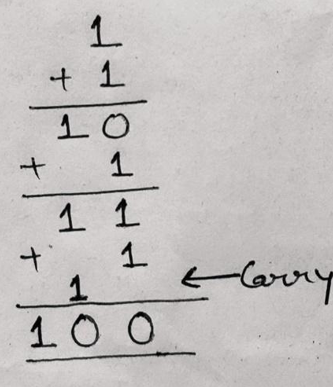### Example 3: Perform (110)2 + (111)2 + (101)2

#### Solution

Using the rules provided above, sum operation can be performed as: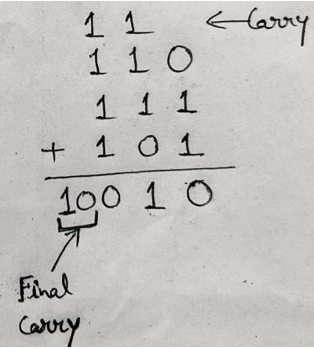Verification:

We can verify our result as (110)2=(6)10, (111)2=(7)10, (101)2= (5)10 and (10010)2= (18)10. So when we will add 6 + 7 + 5 =18, which we are getting as our answer.

## 2) Binary Subtraction

The binary subtraction is performed like decimal subtraction, the rules for binary subtraction are:

ABDifferenceBorrow
0000
0111
1010
1100

### Example 1: Subtract (10)2 from (1001)2

#### Solution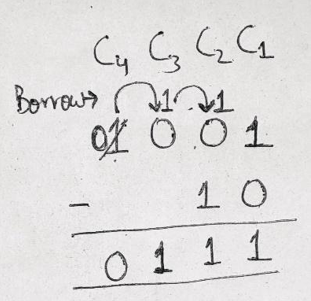In column C2, 1 can't be subtracted from 0 so, we have to borrow 1 from column C3, but C3 also has a 0, so 1 must be borrowed from column C4, the 1 borrowed from column C4 becomes 10 in column C3, now keeping 1 in column C3 bringing the remaining 1 to column C2 which becomes 10 in column C2 thus 10 – 1= 1 in column C2.

In column C3, 1 – 0 = 1

In column C4, 1 after providing borrow 1 is reduced to 0.

Therefore, (1001)2 – (10)2 = (111)2

### Example 2: Subtract (111.111)2 from (1010.01)2

#### Solution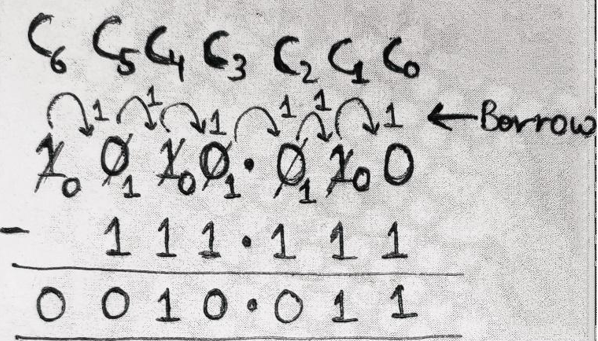In Column C0, 1 can't be subtracted from 0, so we have to borrow 1 from column C1, which becomes 10 in column C0, thus 10 – 1 = 1,

In column C1, after providing borrow 1 to C0, C1 is reduced to 0. Now 1 can't be subtracted from so borrow 1 from C2, but it is also 0, so borrow 1 from C3 which is also 0, so borrow 1 from C4, reducing column C4 to 0. Now, this 1 borrowed from column C4 becomes 10 in column C3, keep 1 in the column C3 and bring other 1 to column C2, which makes column C2 as 10 now again bring 1 from C2 to C1, which reduces C2 to 1 and makes C1 as 10.

Thus, In Column C1, 10 – 1 = 1

In Column C2, 1 – 1 = 0

In Column C3, 1 – 1 = 0

In Column C4, we now have 1 to be subtracted from 0 which is not possible so we will borrow 1 from Column C5, but Column C5 has a 0 so borrow 1 from C6 making C6 to be 0 and bring it to C5 which makes it 10 in C5, keep 1 in C5 and bring the other 1 to C4 which makes C4 as 10 thus

In column C4, 10 – 1 = 1

In column C5, 1 – 1 = 0

In column C6, 0 – 0 = 0

Hence, the result is (1010.01)2 – (111.111)2 = (0010.011)2